Home > CCA2 > Chapter 12 > Lesson 12.1.2 > Problem12-29

12-29.
1. For each of the following equations, find the solutions that lie in the domain 0 ≤ x < 2π. Homework Help ✎

1. 2 sin (x) − 1 = 0

2. 2 cos (x) = −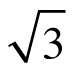3. 2 sin(x) =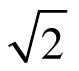4. cos(x) = 1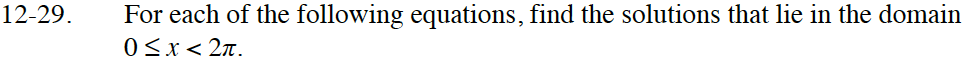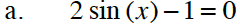$\text{For the equation to be true, sin(\textit{x}) must =}\ \frac{1}{2}.$

You can either draw a unit circle to find all possible solutions, or solve the equation graphically.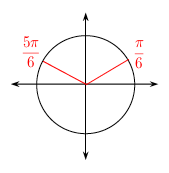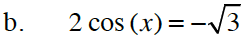In what quadrants is cos(x) negative?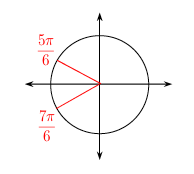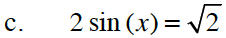$\text{When does sin(\textit{x}) = } \frac{\sqrt{2}}{2}?$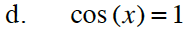Picture a unit circle. At what angle is the adjacent side the same length as the hypotenuse?